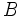# Intransitivity of preferences

Intransitivity of preferences is a phenomenon where a person, given three alternatives$A$,$B$, and$C$, prefers$B$ to$A$,$C$ to$B$, and$A$ to$C$.